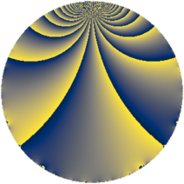# Properties

 Label 287.2.fLevel $287$ Weight $2$ Character orbit 287.f Rep. character $\chi_{287}(50,\cdot)$ Character field $\Q(\zeta_{4})$ Dimension $40$ Newform subspaces $1$ Sturm bound $56$ Trace bound $0$

# Related objects

## Defining parameters

 Level: $$N$$ $$=$$ $$287 = 7 \cdot 41$$ Weight: $$k$$ $$=$$ $$2$$ Character orbit: $$[\chi]$$ $$=$$ 287.f (of order $$4$$ and degree $$2$$) Character conductor: $$\operatorname{cond}(\chi)$$ $$=$$ $$41$$ Character field: $$\Q(i)$$ Newform subspaces: $$1$$ Sturm bound: $$56$$ Trace bound: $$0$$

## Dimensions

The following table gives the dimensions of various subspaces of $$M_{2}(287, [\chi])$$.

Total New Old
Modular forms 60 40 20
Cusp forms 52 40 12
Eisenstein series 8 0 8

## Trace form

 $$40q + 4q^{3} - 36q^{4} + 8q^{6} + O(q^{10})$$ $$40q + 4q^{3} - 36q^{4} + 8q^{6} - 32q^{10} - 8q^{11} + 16q^{12} + 16q^{13} - 8q^{15} + 28q^{16} + 20q^{17} - 12q^{18} - 20q^{19} + 4q^{22} + 16q^{23} - 12q^{24} - 40q^{25} - 20q^{26} - 20q^{27} - 12q^{29} + 4q^{30} + 32q^{34} + 4q^{35} - 16q^{38} + 64q^{40} + 16q^{41} + 32q^{42} + 8q^{44} + 72q^{45} - 24q^{47} - 40q^{48} - 64q^{51} - 96q^{52} + 8q^{53} + 52q^{54} - 8q^{55} - 88q^{57} - 36q^{58} + 48q^{59} + 52q^{60} - 8q^{63} - 84q^{64} - 44q^{65} + 56q^{66} + 40q^{67} - 60q^{68} + 28q^{69} - 8q^{70} + 20q^{71} + 80q^{72} - 20q^{75} - 4q^{76} + 12q^{78} - 12q^{79} + 16q^{81} - 52q^{82} + 40q^{83} + 8q^{85} + 80q^{86} + 96q^{88} - 8q^{89} - 20q^{92} - 64q^{93} + 52q^{94} + 68q^{96} - 60q^{97} - 4q^{98} - 36q^{99} + O(q^{100})$$

## Decomposition of $$S_{2}^{\mathrm{new}}(287, [\chi])$$ into newform subspaces

Label Dim. $$A$$ Field CM Traces $q$-expansion
$$a_2$$ $$a_3$$ $$a_5$$ $$a_7$$
287.2.f.a $$40$$ $$2.292$$ None $$0$$ $$4$$ $$0$$ $$0$$

## Decomposition of $$S_{2}^{\mathrm{old}}(287, [\chi])$$ into lower level spaces

$$S_{2}^{\mathrm{old}}(287, [\chi]) \cong$$ $$S_{2}^{\mathrm{new}}(41, [\chi])$$$$^{\oplus 2}$$